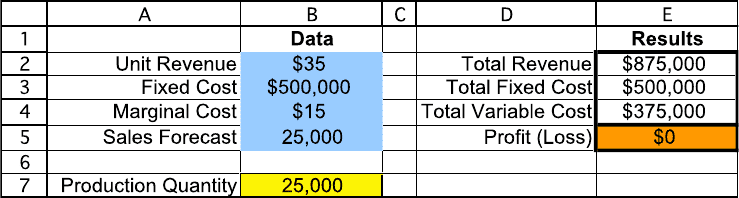Textbook Notes (280,000)
CA (170,000)
UOttawa (6,000)
Chapter 1

# ADM2302 Chapter 1: 1. Week 1 Extra Problems Solution

Department
Course Code
Professor
Rim Jaber
Chapter
1

This preview shows half of the first page. to view the full 3 pages of the document.1
Week 1 Extra Problems Solution
CHAPTER 1
INTRODUCTION
Problems
1.1
If Q units are produced per month, then
Monthly Profit = \$0 {if Q = 0} and \$20,000 + (\$20 \$10)Q {if Q > 0}.
Break-even point = \$20,000 / (\$20 \$10) = 2000, so it will be profitable
to produce if Q > 2000.
1.3
a) Let Q be the number of units produced and sold. Then Monthly Profit
= \$0 {if Q = 0} and \$500,000 + (\$35 \$15)Q {if Q > 0}.Break-even
point = \$500,000 / (\$35 \$15) = 25,000.
b) Let Q be the number produced and s the number that can be sold.
Then
Profit = [0 if Q = 0] and [-\$500,000 + \$35 * MIN(Q, s) \$15Q if Q > 0].
c)
d) Q s.
1.4
a) \$30,000,000
b) \$1,666.67
c) \$1,333.33